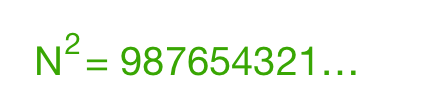# Perfect Square in 987654321...Find the smallest positive integer $N$ such that $N^2$ starts with 987654321.

Note: If you use a calculator for this problem, it can be solved in under a minute. There is no need to do extensive trial and error. You could push a maximum of 100 buttons (inclusive of each digit).

×